# Addition and Subtraction of Decimals

Addition and subtraction of decimals are a bit complex as compared to performing the operations on natural numbers. Before we learn how to add or subtract any two or more than two given decimals, first, let us understand decimals. The decimal number is used to represent a number with greater precision than integers or whole numbers. It uses a dot in between numbers, which is said to be a decimal point. Decimals are nothing but the simplified version of fractions. Check problems based on decimal fractions here.

For example, when we have to divide three pies among 4 kids, we cannot represent the pies received by each kid in terms of integers alone, as each kid has received pies that lie between 0 and 1. To deal with other similar problems, the concept of decimal was introduced. Thus, the idea of addition, multiplication, division and subtraction of decimals is an important topic. Let us say two friends are contributing to buy a cricket ball that costs Rs. 20. One of them has Rs. 7.50, and the other has Rs. 18.50. To know how much money each will have to contribute and how much they will be left with, they must understand the concept of addition and subtraction of decimals.

As we know numbers can be added, subtracted, multiplied and divided. However, all these type of operations can be easily performed on natural numbers. In case of decimals, addition can be done in a specific way. The addition of decimals involves several steps.

Addition of decimals is performed using the following steps:

Step 1: The numbers are first padded with zero depending upon the maximum digits present after the decimal for any of the numbers.

For example, while adding 3.456 to 7.1, since the number 3.456 has the number of digits after the decimal, the padding is done according to 3.456. Since 3.456 has 3 digits after the decimal, we pad two zeros after 1. So, 7.1 to three places is 7.100.

Step 2: The numbers are lined up vertically along with each other as given in the below figure.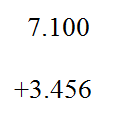Step 3: Finally, add the decimal numbers similar to integers and place the decimal point accordingly.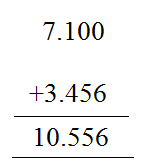Let us understand the concept more clearly with the help of the following examples:

Let us see some examples of adding decimals.

Example 1: Addition 1.091 + 1.33.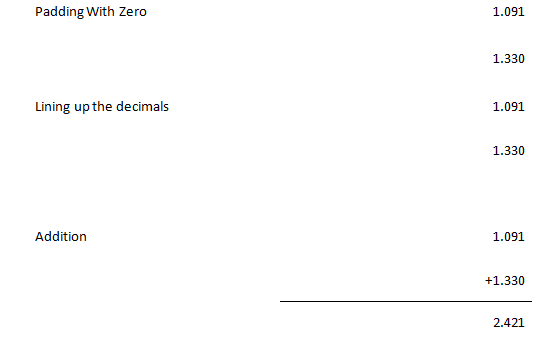Example 2: Addition 0.0075 + 5.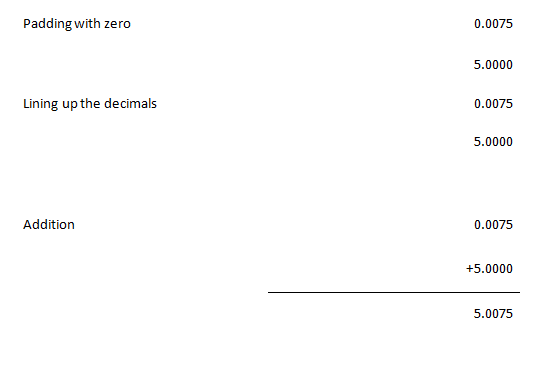Example 3: Add 9.1, 3.22, and 0.66.

Solution:

Given, 9.1, 3.22 and 0.66.

As we can see, 9.1 has only one digit after the decimal but 3.22 and 0.66 have two digits.

Hence, we can write 9.1 as 9.10

Now add all the three decimals.

9.10

+3.22

+0.66

——–

12.98

——

## Subtraction of decimals

Subtraction of decimals involves the subtraction of the decimal number with a small whole number part from the decimal number with a greater whole number part. However, we need to follow certain rules while performing the subtraction on decimals.

### How to Subtract Decimals?

Subtraction of decimals is performed using the following steps:

Step 1: The numbers are first padded with zero depending upon the maximum digits present after the decimal for any of the numbers.

For example, while subtracting 3.456 from 7.1, since the number 3.456 has more digits after the decimal, the padding is done according to 3.456. Since 3.456 has 3 digits after the decimal, we pad 7.1 to three places as 7.100.

Step 2: The numbers are lined up vertically along with each other as shown below.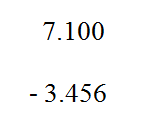Step 3: Finally, subtract the decimal numbers similar to integers and place the decimal point accordingly.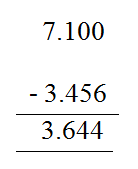### Examples on Decimal Subtraction

Let us understand the concept more clearly with the help of the following examples:

Example 1: Subtraction: 7.304 – 1.15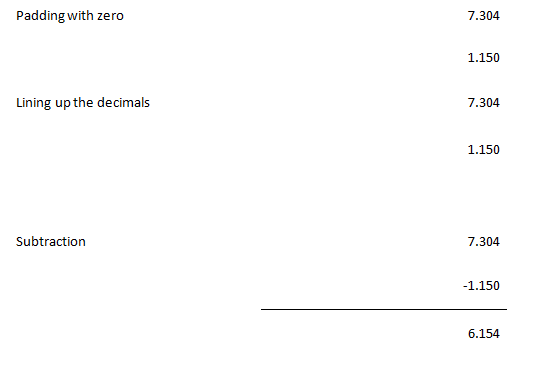Example 2: Subtraction 4.1 – 0.94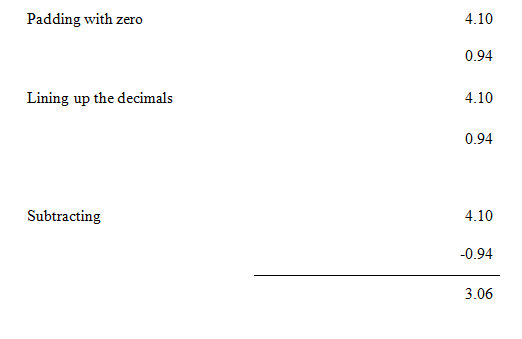### Practice Questions

Try solving the following practice problems to get a thorough understanding of the addition and subtraction of decimal numbers.

1. Add the decimals: 84.956 and 210.28163.
2. Subtract 54.12 from 64.2.
3. Subtract 72.3261 from 80.

## Frequently Asked Questions – FAQs

Q1

### What is addition and subtraction of decimals?

The addition of decimals is similar to the addition of numbers but these numbers contain decimal points. The subtraction of decimals is the process of subtracting a decimal number with a smaller whole number part from the decimal number with a greater whole number part.
Q2

### How to do the addition of decimals?

The addition of decimals involves the following steps:
Step 1: The numbers are first padded with zero depending upon the maximum digits present after the decimal for any of the numbers.
Step 2: The numbers are lined up vertically along with each other.
Step 3: Finally, add the decimal numbers similar to integers and place the decimal point accordingly.
Q3

### How to do subtraction of decimals?

The subtraction of decimals involves the below given steps:
Step 1: The numbers are first padded with zero depending upon the maximum digits present after the decimal for any of the numbers.
Step 2: The numbers are lined up vertically along with each other.
Step 3: Finally, subtract the decimal numbers similar to integers and place the decimal point accordingly.
Q4

### How do you line up decimal numbers in addition and subtraction?

In addition and subtraction of decimals, the given decimals numbers should be lined up with each other with respect to the decimal point.
Q5

### How to subtract a decimal number from a whole number?

To subtract a decimal number from a whole number, we must add the decimal point to the whole number and add zeros as many as required depending on the given decimal number. Suppose, to subtract 6.743 from 9, we must write 9 as 9.000 and then subtract 6.743 from 9.000.